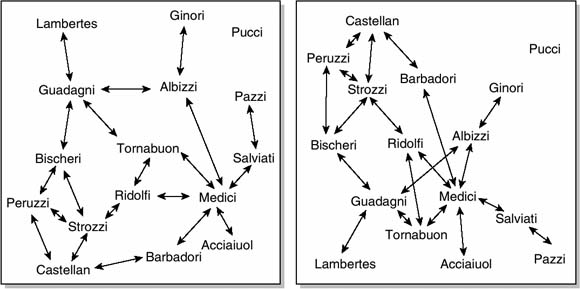Skip to main content Skip to Search Box

Definition: algorithm from Britannica Concise Encyclopedia

Procedure that produces the answer to a question or the solution to a problem in a finite number of steps. An algorithm that produces a yes or no answer is called a decision procedure; one that leads to a solution is a computation procedure. A mathematical formula and the instructions in a computer program are examples of algorithms. Euclid’s Elements (c. 300 bc) contained an algorithm for finding the greatest common divisor of two integers. Manipulation of lists (searching for, inserting, and removing items) can be done efficiently by using algorithms.

Event: algorithm

Keywords: algorithm

Summary Article: Algorithm
from Encyclopedia of Survey Research Methods

Algorithm is a computer science term for a way of solving a problem, and it also refers to the instructions given to the computer to solve the problem. The study of algorithms is central to computer science and is of great practical importance to survey data analysis because algorithms are used in statistical programs.

An algorithm can be thought of as any step-by-step procedure for solving a task. Imagine five playing cards face down on a table and the task of sorting them. Picking them up one at a time with the right hand and placing them in the left hand in their proper place would be one way to solve this task. This is an algorithm, called insertion sort in computer science.Two possible depictions of the same network data

It is worth noting the subtle distinction between the concept of algorithm and the concept of a method or of a technique. For example, a method would be least squares; matrix inversion would be a technique used therein; and LU decomposition and Strassen’s algorithm would be alternative algorithms to accomplish matrix inversion. A single data analysis method may use more than one algorithm.

It is impossible to write statistical software without using algorithms, so the importance of algorithms to survey data analysis is assured. However, user-friendly statistical software packages eliminate the need for end users to construct their own algorithms for most tasks. Nonetheless, at least a basic understanding of algorithms can be useful to survey researchers. For example, maximum likelihood methods can use an initial estimate as a starting point, and in some cases failure to converge may be remediated by trivially altering the initial estimate. Without some familiarity of the underlying algorithm, a researcher may be stuck with a nonconverging function.

Another setting where some knowledge of algorithms is useful is shown in Figure 1, which illustrates two possible depictions of the exact same network data. The left panel uses the multi-dimensional scaling algorithm and the right uses simulated annealing. The data are identical, which may be verified by observing who is connected to whom, but the appearance of the graphs is different. Algorithms are important here, because interpretation of the network data is affected by the appearance of the graph, which is affected in turn by the choice of algorithm. Whereas in many cases different algorithms will produce the same result but differ in speed (i.e., computing time), in this case different algorithms produce different results.

The term algorithm is sometimes used more broadly to mean any step-by-step procedure to solve a given task, whether or not a computer is involved. For instance, matching historical records from more than one archival source can be done by hand using an algorithm. Moreover, it is not only the analysis of survey data that uses algorithms, but also in many cases in the collection of the data an algorithm may be used to select clusters in a complex sample survey design.

Further Readings
• ; ; ; (2001). Introduction to algorithms (2nd ed.). Cambridge: MIT Press.
• (1997). Fundamental algorithms: The art of computer programming (3rd ed., Vol. 1). Reading, MA: Addison-Wesley.
• ; ; ; (2007). Numerical recipes: The art of scientific computing (3rd ed.). Cambridge, UK: Cambridge University Press.

•Copyright © 2008 by SAGE Publications, Inc.

### Related Articles

##### Full text Article Algorithm
The Johns Hopkins Guide to Digital Media

The term algorithm, most commonly associated with computer science, may be used for any effective procedure that reduces the solution of a problem t

##### Full text Article Algorithm
Key Concepts in Teaching Primary Mathematics

DEFINITION An algorithm is a standard procedure that involves a number of steps, which, if followed correctly, can be relied upon to lead to...

##### Full text Article algorithm
Word Origins

[13 century] Algorithm comes from the name of an Arab mathematician, in full Abu Ja far Mohammed ibn-Musa al-Khwarizmi (c. 780-c. 850), who...

See more from Credo